# 3.多變數線性迴歸 （Linear Regression with multiple variable）

## 3.1 多維特徵(Multiple Features)

• n 代表特徵的數量
• x(i)代表第 i 個訓練例項,是特徵矩陣中的第 i 行,是一個向量(vector)。
• x(i)j代表特徵矩陣中第 i 行的第 j 個特徵,也就是第 i 個訓練例項的第 j 個特徵。

hθ=θ0+θ1x+θ2x+...+θnx.

θ=θ0θ1θn
X=x0x1xn

hθ=θTX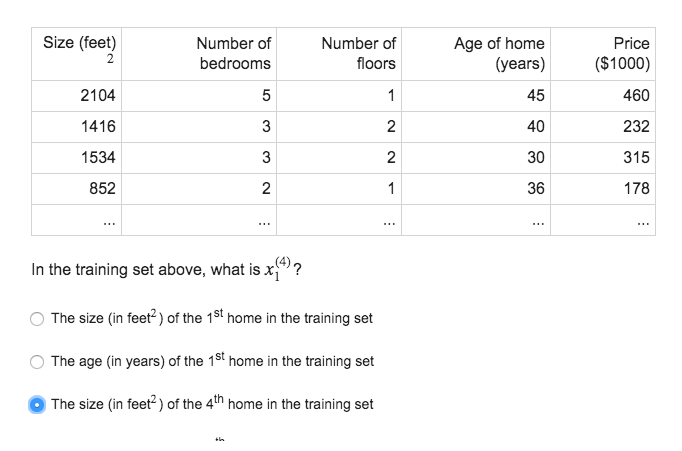## 3.2 多變數梯度下降(Gradient descent for multiple variables)

cost function :

J(θ)=12m1m(hθ(x(i))y(i))2

θj:=θjαθjJ(θ0,θ1)
=θjα1m1m(hθ(x(i))y(i))x(i)j

theta=thetaalpha/mX(Xthetay);## 3.3 特徵縮放(feature scaling)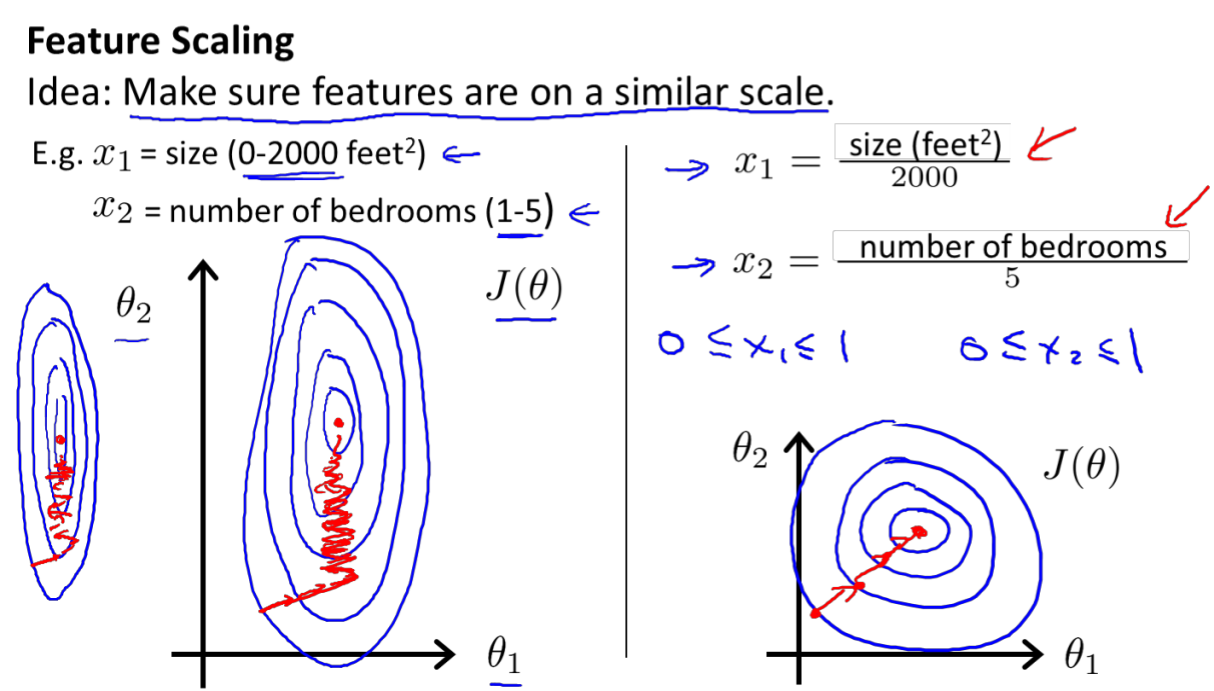mean normalization

xn=xnμnsn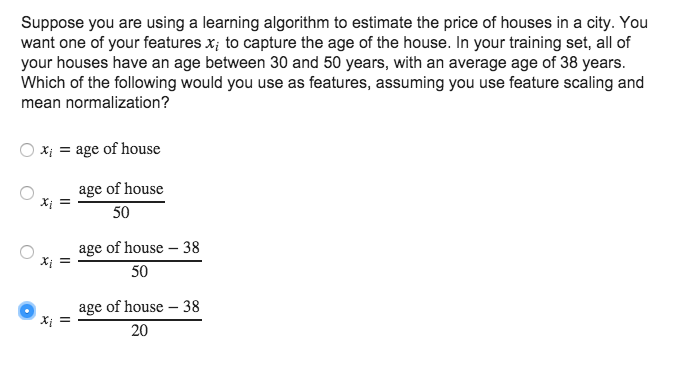## 3.4 學習率(Learning rate)

1. 確保梯度下降working correctly
繪製迭代次數和代價函式的圖表來觀測演算法在何時趨於收斂。下降說明正常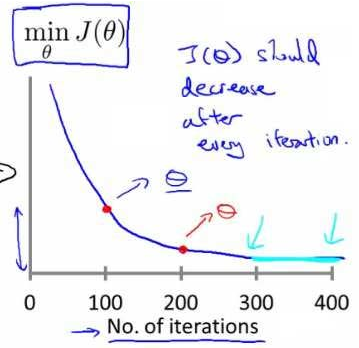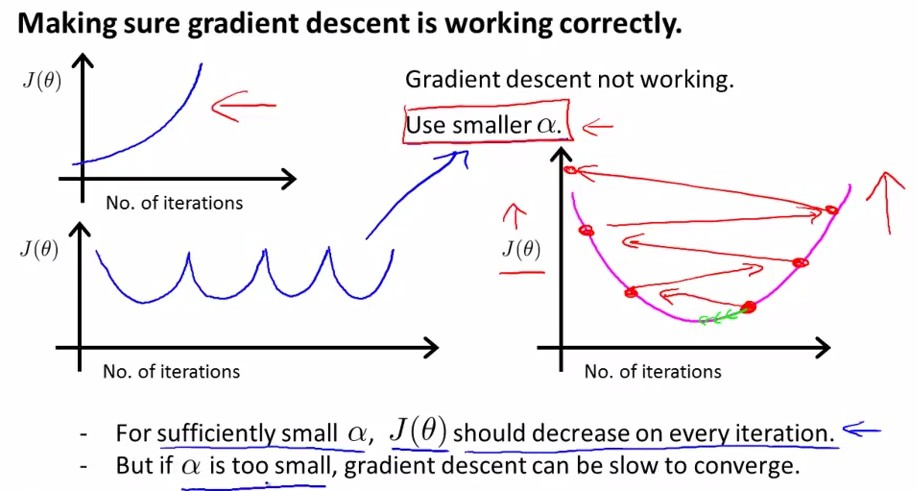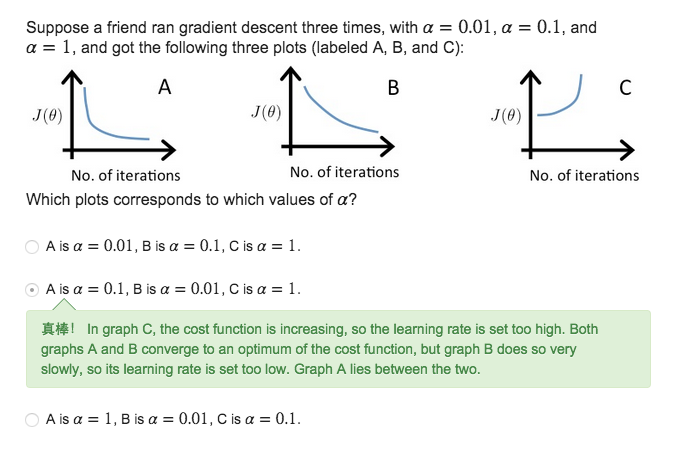2.如何選取 α

We recommend trying values of the learning rate α on a log-scale, at multiplicative steps of about 3 times the previous value
α=…,0.001,0.01,0.1,1,…
α=…,0.001,0.03,0.01,0.03,0.1,0.3,1,…